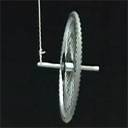# Angular Momentum conceptual question

• brushman

#### brushman

I'm having trouble understanding angular momentum in general.

Given the bicycle wheel gyroscope experiment:Is my line of thinking correct?

The tension in the string equals the mass of the bike wheel. This force alone is holding the bike wheel up.

The wheel is spinning, producing an angular momentum L directly out of the center of the wheel, parallel to the axis of rotation of the wheel. This momentum must be conserved. Only a torque can change this angular momentum.

The only torque is that which is a result of gravity. This torque is the cross product of the radius (the trick bars) and the weight, mg. By the right hand rule, the torque is a vector perpendicular to the angular momentum, and in the same plane. Thus, this torque causes the wheel to rotate around the string.

The wheel doesn't fall down because mg = tension. For the wheel to fall down, there would need to be a torque vector pointing downward. There is none.

In other words, if the wheel were to fall down, the angular vector of the spinning wheel L would change. Thus, there would have to be a torque to change the momentum in that direction, but there is no torque.

The angular momentum L always follows the torque, because torque is just change in momentum over time.

If the tire were spinning the opposite direction, the angular momentum L would be the opposite direction, the torque would be the opposite direction, and so the wheel would precess (rotate around the string) in the opposite direction.

hi brushman!(and btw, no, i have no idea why that image didn't work)
The tension in the string equals the mass of the bike wheel. This force alone is holding the bike wheel up.

yes, except the string isn't quite vertical, so the tension will be slightly less.for that reason, you have to be careful which point you take torque an angular momentum about …

you can't choose the bottom of the string since it's moving, so you choose the top of the string
The wheel is spinning, producing an angular momentum L directly out of the center of the wheel, parallel to the axis of rotation of the wheel. This momentum must be conserved. Only a torque can change this angular momentum.

The only torque is that which is a result of gravity.

yes, the only other external force is the tension, which has zero torque about the chosen point
This torque is the cross product of the radius (the trick bars) and the weight, mg. By the right hand rule, the torque is a vector perpendicular to the angular momentum, and in the same plane.

that's right … the torque is horizontal and "tangential"

but from here on, you're oversimiplifying …

not any old torque will do, it has to be exactly right (compared with the magnitude of the angular momentum), otherwise the gyroscope will start falling (or rising) as well as precessing …

all the horizontal torque tells you is the the angular momentum will change slightly "tangentially" …

if it changes just right, then the angular momentum will stay "radial" (and move uniformly in a circle), otherwise it will start to have a "tangential" component, rotating the gyroscope up or down